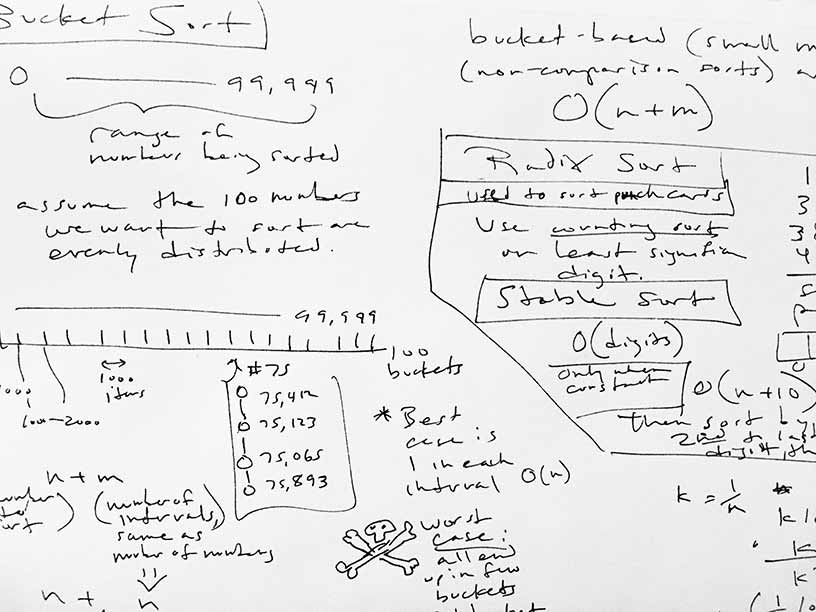I put on my sorting hat and watched many, many videos on sorting.

The videos covered:

• bubble sort
• selection sort
• insertion sort
• heap sort
• merge sort
• quicksort
• counting sort

There are several more sorting algorithms I could have gone into, but I had to stop somewhere. I'd like to learn about bitonic sort, signature sort, etc. So I added another few videos to the sorting section that I'll get around to.

What I really like about sorting is the terse code needed to do something very powerful. Even though comparison-based sorting theoretically can't do better that O(n log n), that's pretty darn good. Given the right constraints, such as all your keys to sort are the same length and a small k (for digits, 0..9), you can switch to radix sort and rock O(n) sorting.

Want to see some code?

### Merge Sort

Merge sort requires O(n) additional space.

``````namespace jw {

void merge(int numbers[], int low, int mid, int high) {
// temp array for holding sorted items
int b[high - low - 1];
int i = low;
int j = mid + 1;
int k = 0;

// merge items from list in order
while (i <= mid && j <= high) {
if (numbers[i] <= numbers[j]) {
b[k++] = numbers[i++];
} else {
b[k++] = numbers[j++];
}
}

// copy the remaining items to tmp array
while (i <= mid) b[k++] = numbers[i++];
while (j <= high) b[k++] = numbers[j++];

--k;
while (k >= 0) {
numbers[low + k] = b[k];
--k;
}
}

void merge_sort(int numbers[], int low, int high) {
if (low < high) {
int mid = (low + high) / 2;

merge_sort(numbers, low, mid);
merge_sort(numbers, mid + 1, high);
merge(numbers, low, mid, high);
}
}

}  // namespace jw
``````

### Quicksort

Note this uses random pivot selection, and sorts in-place.

``````import random

class QuickSort(object):

def __init__(self, numbers):
self.values = numbers
self.count = len(self.values)

def sort(self):
self.quick_sort(0, self.count - 1)
return self.values

def quick_sort(self, left, right):
if left == right:
return

i = left
j = right

pivot_index = random.randint(left, right)

pivot = self.values[pivot_index]

while i <= j:
while self.values[i] < pivot:
i += 1
while self.values[j] > pivot:
j -= 1
if i <= j:
if i < j:
temp = self.values[i]
self.values[i] = self.values[j]
self.values[j] = temp
i += 1
j -= 1

if left < j:
self.quick_sort(left, j)
if right > i:
self.quick_sort(i, right)
``````

And here are some notes. I take lots of notes.